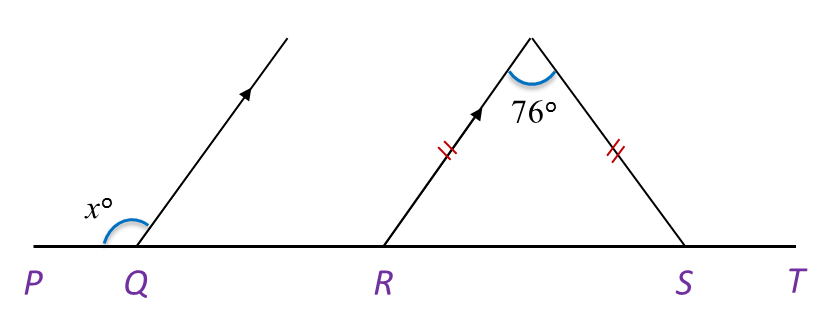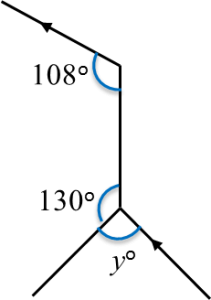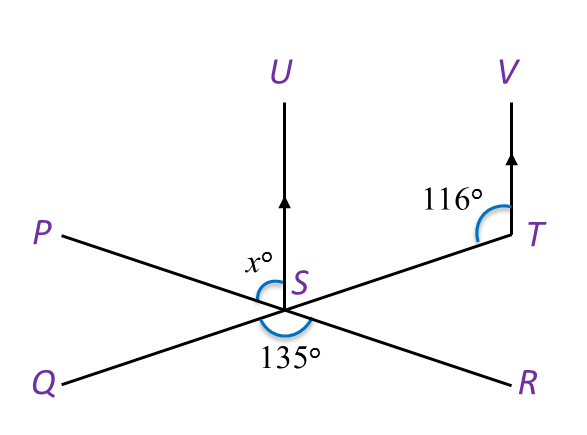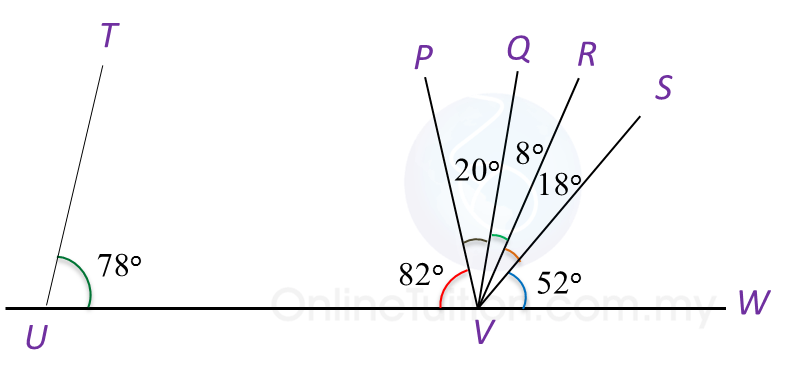# 1.2.2 Angles and Lines II, PT3 Practice

Question 6:
In Diagram below, PQRST is a straight line. Find the value of x.Solution:

Question 7:
In Diagram below, find the value of y.Solution:Question 8:
In Diagram below, PSR and QST are straight lines.Find the value of x.

Solution:
$\begin{array}{l}\angle UST+\angle STV={180}^{o}\\ \angle UST={180}^{o}-{116}^{o}={64}^{o}\\ \angle PST=\angle QSR\\ {x}^{o}+\angle UST={135}^{o}\\ {x}^{o}+\angle UST={135}^{o}\\ {x}^{o}={71}^{o}\\ x=71\end{array}$

Question 9:
In Diagram below, PWV is a straight line.(a) Which line is perpendicular to line PWV?
(b) State the value of  ∠ RWU.

Solution:
(a) SW

(b) ∠ RWU = 13o + 29o + 20o = 62o

Question 10:
In Diagram below, UVW is a straight line.(a) Which line is parallel to line TU?
(b) State the value of  ∠ QVS.

Solution:
(a) QV

(b) ∠ QVS = 8o + 18o = 26o